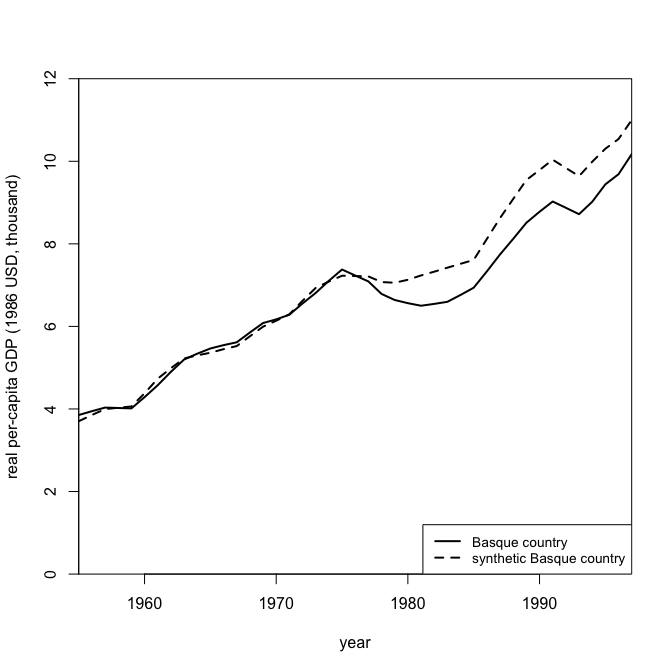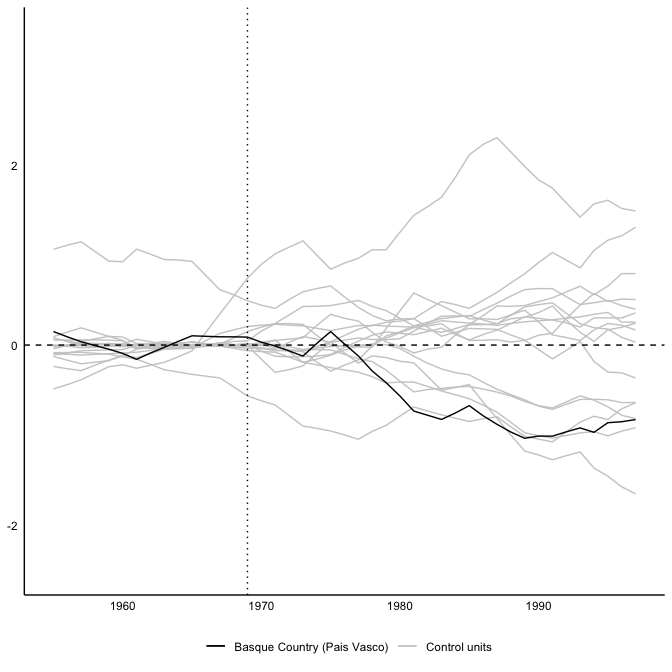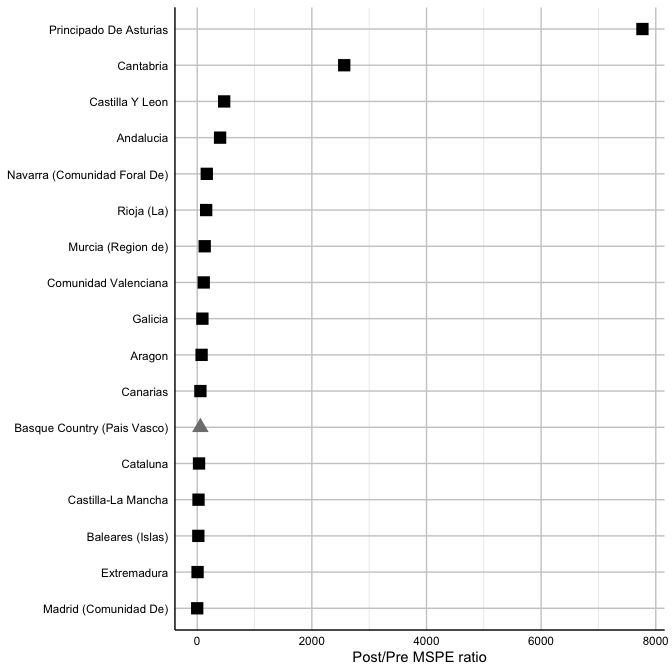# Replicating the Basque Study

## Introduction

In order to demonstrate SCtools it is useful to start with a replication of the cannonical Basque study from Abadie and Gardeazabal (2003).

library(SCtools)
library(Synth)

Now we can load the basque data set.

data("basque")

As per the normal Synth workflow, we need to reformat our data using the dataprep function in which we specify our counterfactuals and our response variables.

dataprep.out <- dataprep(
foo = basque,
predictors = c("school.illit", "school.prim", "school.med",
"school.high", "school.post.high", "invest"),
predictors.op = "mean",
time.predictors.prior = 1964:1969,
special.predictors = list(
list("gdpcap", 1960:1969 ,"mean"),
list("sec.agriculture", seq(1961, 1969, 2), "mean"),
list("sec.energy", seq(1961, 1969, 2), "mean"),
list("sec.industry", seq(1961, 1969, 2), "mean"),
list("sec.construction", seq(1961, 1969, 2), "mean"),
list("sec.services.venta", seq(1961, 1969, 2), "mean"),
list("sec.services.nonventa", seq(1961, 1969, 2), "mean"),
list("popdens",               1969,               "mean")),
dependent = "gdpcap",
unit.variable = "regionno",
unit.names.variable = "regionname",
time.variable = "year",
treatment.identifier = 17,
controls.identifier = c(2:16, 18),
time.optimize.ssr = 1960:1969,
time.plot = 1955:1997)

Now, we can run the SCM algorithm using the synth function.

synth.out <- synth(data.prep.obj = dataprep.out, method = "BFGS")

Synth provides some additional helper functions to extract information from the outputted object including the ability to analyze the outputs:

gaps <- dataprep.out$Y1plot - (dataprep.out$Y0plot %*% synth.out\$solution.w)

And generate plots of the counterfactual:

path.plot(synth.res = synth.out, dataprep.res = dataprep.out,
Ylab = "real per-capita GDP (1986 USD, thousand)", Xlab = "year",
Ylim = c(0, 12), Legend = c("Basque country",
"synthetic Basque country"),
Legend.position = "bottomright")## Extending the Analysis

At this point, SCtools extends the analysis from Synth, While Synth generates an analysis on one configured dataset, SCtools provides the tooling to permute the dataset and generate multiple placebos to test the sensitivity of our SCM output.

placebo <- generate.placebos(dataprep.out = dataprep.out,
synth.out = synth.out, strategy = "multiprocess")

We can then use the plot_placebos to run a placebo test for the findings in Abadie and Gardeazabal (2003).

plot_placebos(placebo)Finally, we can also run the mspe_plot function to run a post/pre MPSE test for that case, and find how unlikely it would be to find by chance the effects identified.

mspe_plot(placebo)